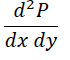# Dtermining dimensions.

pyroknife

## Homework Statement

Let P represent a force and x, y, and z represent distances. Determine the dimensions for each of the quantities listed below.

I attached the problem I need help with. (it's a small picture)

So I'm a bit confused since the it involves both dx and dy.

The dimensions for P is (ML/T^2)
Where M=mass
L=length
T=time

Then I have to differentiate it twice but with two different variables. Since x and y refer to distances and the only distance found in that equation is L.

Would that mean the dimensions for dP/dx = M/T^2 ?

If so, would that mean the answer to the 2nd derivative still be the same answer since they're differentiating between different variables? (I'm not sure if I worded this right, but I remember from Calc III something similar)

On a side note. I took calculus quite a while ago so some of this I might have forgotten.
The picture I provided, that is not equivalent to (dP/dx)*(dP/dy) right?

#### Attachments

•Untitled.png
907 bytes · Views: 342
Last edited: